Home > GC > Chapter 8 > Lesson 8.3.3 > Problem8-110

8-110.
1. the graph of f(x) below. Use the graph to find the following values.Homework Help ✎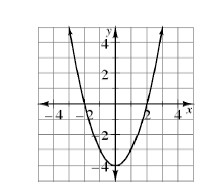1. f(1)

2. f(0)

3. x if f(x) = 4

4. x if f(x) = 0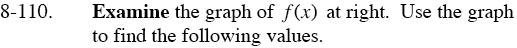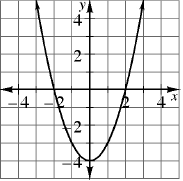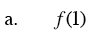f(1) = the value of y on the graph when x = 1.

f(1) = −3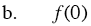Locate x = 0 on the graph. Find the y-coordinate for the point (0, y). This is the value of f(0).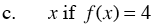f(x) = 4 is the equivalent of y = 4 on the graph.
Therefore, look for the values of x when y = 4.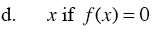Find the values of x when y = 0, so look for the points
(x, 0) on the graph.